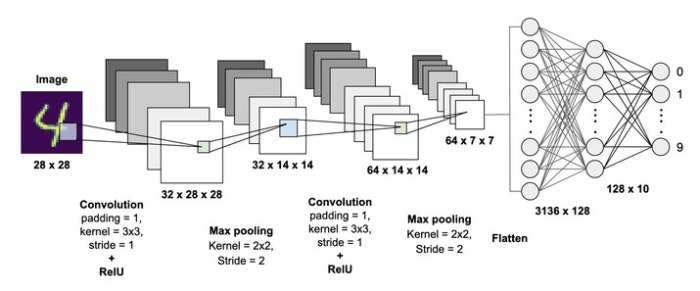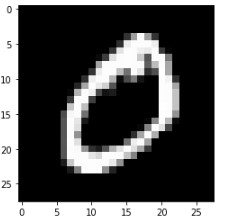# Handwritten Digit Recognition using Neural Network

## Introduction

Handwritten Digit Recognition is a part of image recognition widely used in Computer Vision in Deep learning. Image recognition is one of the very basic and preliminary stages of every image or video−related task in Deep Learning. This article lets an overview of Handwritten Digit Recognition and how Image recognition can be extended to multiclass classification.

Before going ahead let us understand the difference between Binary and Multiclass image classification

## Binary Image Classification

In Binary image classification, the model has two classes to predict from. For example in the classification of cats and dogs.

## Multiclass Image Classification

In Multiclass Image Classification, the model has more than two classes to predict from. For example, in the classification of FasnionMNIST or Handwritten Digit Recognition, we have 10 classes to predict from.

## Handwritten Digit RecognitionThis task is a case of Multiclass image classification where the model predicts one of the digits from 0 to 9 to which the input image belongs.

In the MNIST digit recognition task, we use a CNN network to develop a model to recognize the handwritten digit. We would download the MNIST dataset which consists of a training set of 60000 images and 10000 images for testing. Each image is cropped into 28x28 pixels and handwritten digits are from 0 to 9.

## Implementation using Python

### Example

## Digit Recognition

import keras
from keras.layers import Conv2D, MaxPooling2D
from keras.models import Sequential
from keras import backend as K
from keras.datasets import mnist
from keras.utils import to_categorical
from keras.layers import Dense, Dropout, Flatten
import matplotlib.pyplot as plt
%matplotlib inline

fig = plt.figure
n_classes = 10
input_shape = (28, 28, 1)
batch_size = 128
num_classes = 10
epochs = 10

(X_train, Y_train), (X_test, Y_test) = mnist.load_data()
print("Training data shape {} , test data shape {}".format(X_train.shape, Y_train.shape))

img = X_train

plt.imshow(img, cmap='gray')
plt.show()

X_train = X_train.reshape(X_train.shape, 28, 28, 1)
X_test = X_test.reshape(X_test.shape, 28, 28, 1)

Y_train = to_categorical(Y_train, n_classes)
Y_test = to_categorical(Y_test, n_classes)
X_train = X_train.astype('float32')
X_test = X_test.astype('float32')
X_train /= 255
X_test /= 255
print('x_train shape:', X_train.shape)
print('train samples ',X_train.shape,)
print('test samples',X_test.shape)

model = Sequential()

history = model.fit(X_train, Y_train,batch_size=batch_size,epochs=epochs,verbose=1,validation_data=(X_test, Y_test))

output_score = model.evaluate(X_test, Y_test, verbose=0)
print('Testing loss:', output_score)
print('Testing accuracy:', output_score)


### Output

Training data shape (60000, 28, 28) , test data shape (60000,)x_train shape: (60000, 28, 28, 1)
train samples  60000
test samples 10000
Epoch 1/10
469/469 [==============================] - 13s 10ms/step - loss: 2.2877 - accuracy: 0.1372 - val_loss: 2.2598 - val_accuracy: 0.2177
Epoch 2/10
469/469 [==============================] - 4s 9ms/step - loss: 2.2428 - accuracy: 0.2251 - val_loss: 2.2058 - val_accuracy: 0.3345
Epoch 3/10
469/469 [==============================] - 5s 10ms/step - loss: 2.1863 - accuracy: 0.3062 - val_loss: 2.1340 - val_accuracy: 0.4703
Epoch 4/10
469/469 [==============================] - 5s 10ms/step - loss: 2.1071 - accuracy: 0.3943 - val_loss: 2.0314 - val_accuracy: 0.5834
Epoch 5/10
469/469 [==============================] - 4s 9ms/step - loss: 1.9948 - accuracy: 0.4911 - val_loss: 1.8849 - val_accuracy: 0.6767
Epoch 6/10
469/469 [==============================] - 4s 10ms/step - loss: 1.8385 - accuracy: 0.5744 - val_loss: 1.6841 - val_accuracy: 0.7461
Epoch 7/10
469/469 [==============================] - 4s 10ms/step - loss: 1.6389 - accuracy: 0.6316 - val_loss: 1.4405 - val_accuracy: 0.7825
Epoch 8/10
469/469 [==============================] - 5s 10ms/step - loss: 1.4230 - accuracy: 0.6694 - val_loss: 1.1946 - val_accuracy: 0.8078
Epoch 9/10
469/469 [==============================] - 5s 10ms/step - loss: 1.2229 - accuracy: 0.6956 - val_loss: 0.9875 - val_accuracy: 0.8234
Epoch 10/10
469/469 [==============================] - 5s 11ms/step - loss: 1.0670 - accuracy: 0.7168 - val_loss: 0.8342 - val_accuracy: 0.8353
Testing loss: 0.8342439532279968
Testing accuracy: 0.8353000283241272


## Conclusion

In this article, we have studied how we perform recognition of Handwritten Digit using a Neural Network.

Updated on: 30-Dec-2022

627 Views